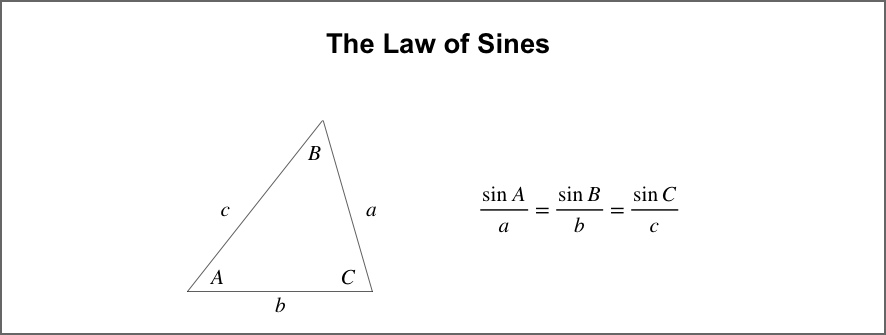It’s math time! Or, at least, designer cheat-sheet time. This week on Tutorial Tuesday we’ll be giving you the answers you need for deducing side lengths and angles of non-right triangles in your 3D designs. If you’re using Fusion 360 or even more professional design software, then depending on how you created your 3D model, you might be able to get all the measurements you need from the design software itself. But sometimes your software programs can’t rescue you when you really need to know exactly how long a slanted line segment is, or how big an angle must be, given other information about your design.

A few months ago we talked about ways to find lengths and angle measures of right triangles given partial information in Tutorial Tuesday 28: Everything You Always Wanted to Know About X, But Were Afraid to Ask (Part 1). But what if the triangles in your design aren’t right triangles? That is, what if your triangles don’t have any 90-degree “right” angles at all? It turns out that math can still come to the rescue…

### Law of Sines

If you know any two angles and any one side length of a triangle, then mathematics can help you determine all of the remaining side and angle measurements for that triangle. To find the third angle, you can use the fact that the sum of all the angles of a triangle is always 180 degrees. To find the lengths of the other sides of the triangle, you can use what’s known as the Law of Sines. Here’s what it looks like (don’t worry, if you don’t remember your trigonometry then you can just think of the “sin” function as a button on your calculator):This can be useful if you want to solve classic mathematical word problems in school, but it’s also useful for a lot of 3D designs, for example if three points on a ring design formed a triangle where you knew one length and two angles of the triangle, but needed two know one of the remaining lengths. Below is an illustration of such an example; imagine that you know the length c and the angle measures A and B, and need to figure out the length X.The Law of Sines comes in handy whenever you know two angles and any side of a non-right triangle. If instead you know two sides and an angle between them, you might want to check out the Law of Cosines instead. If you’d like to get deeper into the mathematics of how to use the Law of Sines, and why it works, check out the Khan Academy video Solving for a side with the Law of Sines.

What math facts do you want to know for your 3D design work? Send us a note and we’ll incorporate your questions into a future Tutorial Tuesday post!

Cover image: Two copies of 8 bit Mario Block by 8 bit Nirvana